# Erroneous decoding, probability of

(diff) ← Older revision | Latest revision (diff) | Newer revision → (diff)

One of the possible measures for characterizing the accuracy of information reproduction over a communication channel (see also Information, exactness of reproducibility of). For the transmission of informationfrom a source which hasdifferent possible values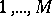with probability distribution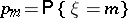,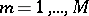, a certain communication channel is used. Then for fixed methods of coding and decoding (see also Information, transmission of), the probability of erroneous decoding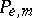,, is defined by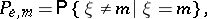whereis the decoded message at the channel output. The variable quantityis called the mean probability of erroneous decoding. The study of the optimal probability of erroneous decoding,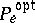, defined by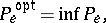is of particular interest. The infimum is taken over all possible methods of coding and decoding. Owing to the difficulty of obtaining an accurate expression for, which is caused by the fact that optimal coding methods are usually unknown, the study of the asymptotic behaviour ofwhen the length of the message in the channel increases is of interest. More precisely, the following situation is studied. Let a segment of lengthin a communication channel with discrete time be used, and put. The asymptotic behaviour ofhas to be studied whenand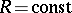(this means that the length of the message increases, while its transmission rate remains constant). If the channel is a homogeneous memoryless channel with discrete time and if the spacesand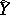of values of the components of the input and output signals are finite, then the following upper and lower bounds for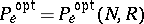are known:(1)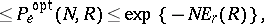where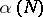and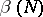tend to zero with increasing, while the functionsand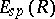are defined as follows:(2)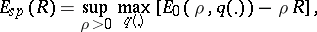(3)

whereHere,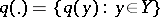is an arbitrary probability distribution on,,,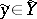, while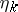and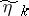,, are the components of the input and output signals of the memoryless channel being considered. It is known that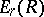and, defined by the formulas (2) and (3), are positive, convex from below, monotone decreasing functions ofwhen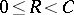, whereis the transmission rate of the channel (cf. Transmission rate of a channel), and that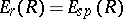when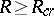; here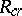is a quantity defined by the transition matrix of the channel and called the critical speed of the given memoryless channel. Thus, for values,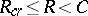, the main terms of the upper and lower bounds (1) forcoincide asymptotically, hence for this range ofthe exact value of the reliability function of the channel,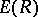, defined by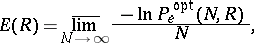is known. For values of,, the exact value offor arbitrary memoryless channels is still unknown (1983), although the bounds (1) can still be improved. The exponential character of decrease ofhas been proved for a very broad class of channels.

How to Cite This Entry:
Erroneous decoding, probability of. Encyclopedia of Mathematics. URL: http://encyclopediaofmath.org/index.php?title=Erroneous_decoding,_probability_of&oldid=14369
This article was adapted from an original article by R.L. DobrushinV.V. Prelov (originator), which appeared in Encyclopedia of Mathematics - ISBN 1402006098. See original article